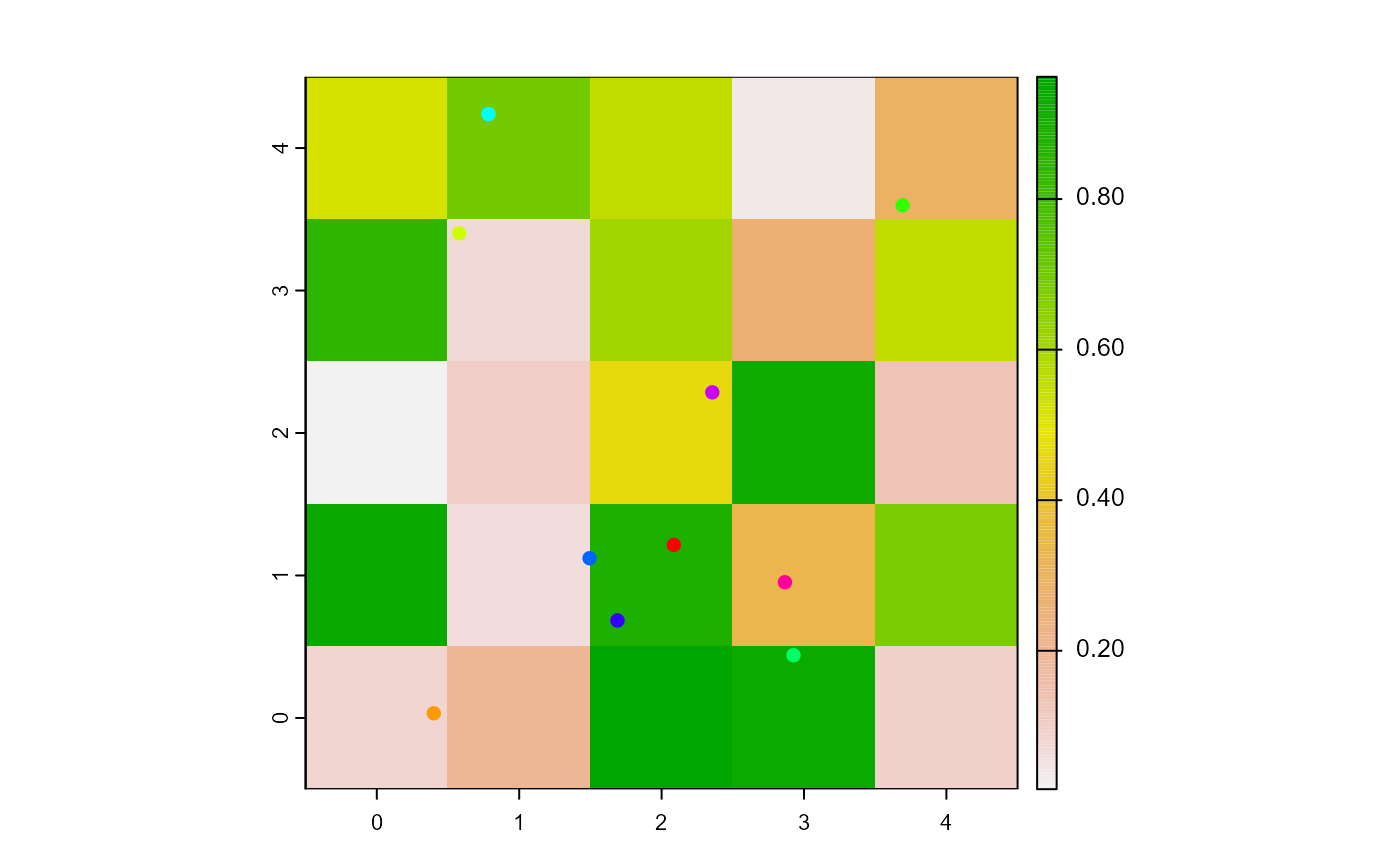Report n random ycor coordinates within the world's extent.

randomYcor(world, n)

# S4 method for worldNLR,numeric
randomYcor(world, n)

## Arguments

world

WorldMatrix or worldArray object.

n

Integer.

## Value

Numeric. Vector of length n of ycor coordinates.

Sarah Bauduin

## Examples

w1 <- createWorld(
minPxcor = 0, maxPxcor = 4, minPycor = 0, maxPycor = 4,
data = runif(25)
)
t1 <- createTurtles(n = 10, coords = cbind(
xcor = randomXcor(world = w1, n = 10),
ycor = randomYcor(world = w1, n = 10)
))
plot(w1)
points(t1, col = of(agents = t1, var = "color"), pch = 16)